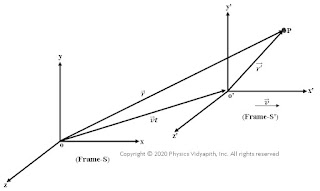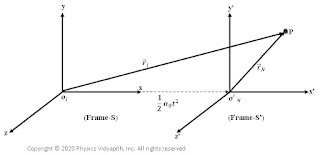### Frame of References (Inertial Frame and Non Inertial Frame)

It is assumed that space is continuous and the motion of particles in space can be described by their position at different instants of time. The position of a particle is known as a point in space. These points are described by the coordinate system in space.

When point (position of particle in space) and the time are taken together then it is called an Event.
The coordinate system of a particle which describe the position of any particle relative to it, then such coordinate system is known as Frame of Reference or System of Reference.

Absolute Space:

The absolute space is those frame of reference relative to which every motion and position should be measured.

Types of Frame of Reference:

According to the motion of particles frame of reference is divided into two categories

1. Inertial frame of reference
2. Non-inertial frame of reference
Inertial frame of reference:

Those unaccelerated frame of reference, in which Newton's first and second laws hold, are called inertial frames.
In an inertial frame, body does not experience any force so according to second law of Newton-

$F=ma \qquad (1)$

$F=0$

so from equation $(1)$

$ma=0$

$a=0$

$\frac{d^2r}{dt^2}=0\qquad (2)$

In component form, above equation, can be written as:

$\frac{d^2x}{dt^2}=0;\:\:\:\frac{d^2y}{dt^2}=0;\:\:\:\frac{d^2z}{dt^2}=0\qquad (3)$

Discussion of Inertial Frame in terms of relative frame of reference:

Let us consider an inertial frame $S$ and another frame $S'$ which is moving with constant velocity $v$ relative to frame $S$. If the position of the origins of the two frames coincide, then in the two frames the position vector of any particle $P$ at any instant $t$ can be related by the following expression-Inertial Frame of Reference

$\overrightarrow{r}=\overrightarrow{oo'}+\overrightarrow{r'}$

$\overrightarrow{r}=\overrightarrow{v}t+\overrightarrow{r'} \qquad \left \{ {\overrightarrow{oo}'=\overrightarrow{v}t} \right \}$

Differentiate the above equation

$\frac{d\overrightarrow{r}}{dt}=\overrightarrow{v}+\frac{d\overrightarrow{r'}}{dt}$

Again differentiate the above equation

$\frac{d^2\overrightarrow{r}}{dt^2}=\frac{d^2\overrightarrow{r'}}{dt^2}$

 $a=a'$

Now we conclude that "If a frame is an inertial frame then all those frames which are moving with constant velocity relative to the first frame are also inertial"

Non-inertial Frame:
Those frames of reference in which Newton's law of inertia does not hold are called non-inertial frames.
All the accelerated and rotating frames are the non-inertial frames of reference.

According to Newton's Second Law, the force $F$ applied on a body of mass $m$ is given by

$F_{i}=ma_{i}\qquad (1)$

Newton's Second Law is not valid when a body of mass $m$ self accelerated and accelerated body will observe the acceleration $a_{N}$. Hence

$F_{i}\neq ma_{N}\qquad (2)$

If no external force is acting on a particle. Even then in the accelerated frame, It will appear that a force is acting on it. This force is called pseudo force or fictitious force. The direction of force is opposite to acceleration.

$F_{o}\neq ma_{o}\qquad (3)$

Discussion of Non-inertial Frame in term of relative frame of reference:

Let us consider, an inertial frame $S$ and another frame $S'$ is moving with an acceleration $a_{0}$ relative to frame $S$.Non-inertial Frame of Reference

$\overrightarrow{r_{i}}=\overrightarrow{r_{N}}+\frac{1}{2}a_{0}t^{2}$

Differentiate the above equation with respect to $'t'$

$\frac{d\overrightarrow{r_{i}}}{dt}=\frac{d\overrightarrow{r_{N}}}{dt}+a_{0}t$

Again differentiate the above equation with respect to $'t'$

$\frac{d^{2}\overrightarrow{r_{i}}}{dt^{2}}=\frac{d^{2}\overrightarrow{r_{N}}}{dt^{2}}+a_{0}$

$a_{i}=a_{N}+a_{0}$

$a_{i}-a_{0}=a_{N}$

$ma_{i}-ma_{0}=ma_{N}$

 $F_{i}+F_{0}=F_{N}$

This formula gives the observed force $F_{N}$ in the accelerated system.